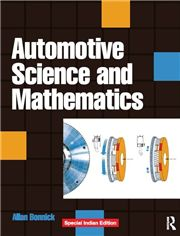# AUTOMOTIVE SCIENCE AND MATHEMATICS, 1/e ALLAN BONNICK-S.CHAND

450.00 405.00

• Mathematics, Arithmetic and Statistics
• Algebra and Formulae
• Graphs
• Geometry and Trigonometry
• Forces
• Levers and Moments
• Work, Energy and Power
• Friction
• Machines
• Velocity and Acceleration - Equations of Motion
• Heat and Temperature
• Heating and Expansion of Gases
• Practical Engine Cycles
• Ideal Engine Cycles
• Fuels, Combustion and Emission Controls
• Vehicle Dynamics - Load Transfer under Acceleration and Braking
• Forces Acting on Vehicle During Cornering and on a Slope – Grade ability
• Electrical Principles
• Electronic Principles